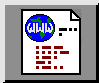## Unfolding the Story of the Folded Dipole

### L. B. Cebik, W4RNLThe folded dipole is a simple antenna to build. However, it has a acquired something of a complex web of correct and incorrect information surrounding it. The points of these notes is to sort out some of the information, with an emphasis upon what it is correct to say about the folded dipole.Fig. 1 shows the essential elements of a folded dipole. It consists of two parallel wires having a constant spacing, S. Each wire has a certain diameter, d1 and d2. The ends of the parallel wires are connected to form a continuous loop. The feedpoint is at the center of the wire having the diameter d1.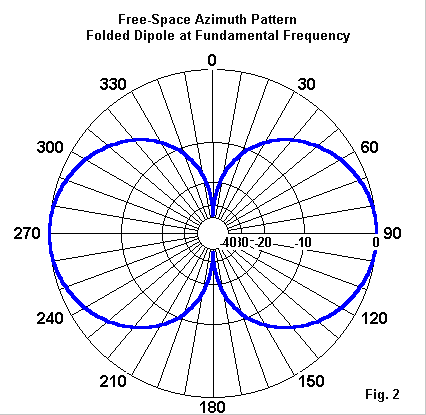We can construct a folded from common materials ranging from house wire to parallel transmission line. With such materials, we can obtain the pattern shown as a free-space azimuth pattern in Fig. 2--the same pattern as a single-wire dipole. The folded dipole is a reliable antenna, meaning that we can get it to work without lots of finicky adjustments. Something about the ease of building an antenna seems to go hand-in-hand with not get a firm grasp on why it works.

In calling the antenna a folded dipole, we should note that the term "dipole" is important to our discussion. "Dipole" is a term that we use as a shorthand for a longer characterization of a single wire antenna. The 1-wire antenna described is a 1/2 wavelength long, resonant, 2-pole antenna. The reference to length is obvious. Being resonant means that the feedpoint impedance will have negligible reactance and hence be purely or close to purely resistive. Having 2 poles means having two transitions from maximum to minimum current--in this case stating at the current maximum located at the center of the antenna.

Now all that we need to deal with is the folded aspect of the antenna. Folding refers not only to the visual appearance of the antenna, but as well to what folding does. Folding a single wire antenna (and thereby doubling the amount of wire needed) creates a combination of an antenna element and an impedance transformer. The same principle has been used with other antenna types. For example, the side-fed rectangle--a good vertically polarized performer for the lower HF bands--has a low feedpoint impedance. Doubling the loop with a cross-over at the far end from the feedpoint raises the impedance of the antenna.

Using antenna transformer techniques to raise the impedance of an antenna does not reduce any losses inherent in the antenna operation. Loss resistances will also be transformed. These losses are not significant with the standard horizontal folded dipole, but have been a major misunderstanding of its cousin, the folded monopole.

### The Folded Dipole as an Impedance Transformer

Our understanding of the folded dipole has been stunted in part by our use of only a special case within the range of possible transformations. By using the same diameter wire for both d1 and d2, we always end up with a 4:1 impedance transformation relative to a single-wire dipole. However, we usually have no idea why this is so. Let's start with the general transformation properties and work our way back to the special case with which we are familiar.

Relative to a single-wire dipole, the feedpoint impedance will be transformed upward by the ratio R according to the following equation: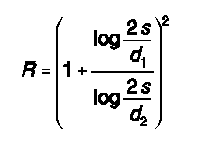where the terms S, d1, and d2 have the meanings shown in Fig. 1.

The log of 2S divided by a wire diameter is a complex quantity that hides some of the consequences of the equation. However, consider that if d1 and d2 are equal diameters, then the division of one log by the other log results in a value of 1. Since 1 plus this value is 2 and the square of 2 is 4, then for wires of equal diameter, the impedance transformation ratio is always 4:1 relative to the impedance of a single wire resonant half-wavelength dipole.

In free space the impedance, the resonant impedance of a single wire resonant half-wavelength antenna that is center-fed is between 71 and 72 Ohms for highly conductive materials like copper. Hence, a folded dipole using equal wire diameters for both wires will be about 284 to 288 Ohms.

Now let's note some other aspects of the equation. There is no rules against using wires of different diameter for d1 and d2. The wire diameter values always occur as divisors (below the division line). Hence, the larger the diameter, the smaller the resulting log term. Therefore, we get the following guidelines (remembering that d1 is the diameter of the fed wire):

• 1. If d1<d2, then R is always >4.
• 2. If d1>d2, then R is always <4.

However, R must always be >1. That is, 1 is the limit of R as the ratio of the two log values goes to zero, which would imply an immensely large value for d2 or an infinitesimally small value for d1. The result is that a folded dipole cannot be used to reduce the feedpoint impedance relative to a single-wire dipole.

So far, we have ignored S. Before taking a log for the numerator and for the denominator of the fraction in the equation, we must divide twice the wire spacing by the wire diameter(s). This results in a different value in the numerator and denominator for every different wire spacing we choose. Hence, the impedance transformation ratio will also change with every change of spacing.

There is one exception to this consequence of spacing. If the two log values result in a value of 1 when we divide one by the other, then the result will always be 4, regardless of the spacing. Hence, for the case where both wires have the same diameter, the feedpoint impedance transformation relative to a single-wire dipole will be 4:1 for any reasonable wire spacing.

There is a limit to how far apart we can place the wires and still have a folded dipole. That limit, however, is considerably farther apart than the limit for having an effective transmission line with confined fields. It also can be a tiny spacing--just enough to prevent a short circuit between the wires.

### Modeling the Folded Dipole

With exceptions that I shall later note, we can use modern antenna modeling software to calculate the properties of folded dipoles of many sorts. For folded dipoles using wires of equal diameter, both MININEC and NEC will yield very accurate results. Remember that the term "modeling" is used in the mathematical sense of calculating antenna properties using equations derived from Poynting Vectors. Hence, the results are very much more accurate than the small rules of thumb formulae we find in many antenna books.

The trick to modeling folded dipoles is to use many segments. The end wires connecting the parallel wires are a limiting factor. In NEC, we want the segment lengths in the parallel wires to be less than a 2:1 ratio in length to the segments in the end wires. In MININEC, we want to use many segments so that the end corners are not mathematically "cut off" in the calculation. So for the models in this exercise, I shall use a frequency of 28.5 MHz with 110 segments along the length of each parallel wire in MININEC to allow a perfectly centered feedpoint. 111 segments is required in NEC. These models will fall well within the calculational constraints of each program type. However, in all cases, the results will apply to bare wire.

As a test case, let's look once more at the question of spacing. We shall use 0.1" diameter copper wires throughout for our initial tests. This diameter is between #12 and #10 AWG wire. I shall present both NEC-2 and MININEC results for comparison. (For reference, the NEC-2 results are from NEC-Win Plus and the MININEC results are from AO 6.5.)

Let's compare the performance of folded dipoles having 3 different spacings. A 1" spacing corresponds to the use of ladder line of common commercial sorts. A 4.14" spacing corresponds to one recommendation that we use a spacing of 1/100 wavelength. Finally, a spacing of 13.8" corresponds to another recommendation that we use a spacing of 1/30 wavelength. In the table below, length refers to the resonant length of the folded dipole, while gain is the free-space gain in dBi. The feedpoint impedance is given in standard series R +/- jX Ohms terms.

```Spacing        Length         Gain           Feedpoint Z
inches         dBi            R +/- jX Ohms
1"
Mininec      196.56"        2.10           288.0 + j0.0
NEC-2        196.93"        2.12           286.6 - j0.0
4.14"
Mininec      193.70"        2.10           288.0 + j0.0
NEC-2        194.20"        2.12           287.0 - j0.1
13.8"
Mininec      186.94"        2.12           287.0 + j0.0
NEC-2        187.40"        2.13           285.8 + j0.0```

Note that the two calculating systems yield resonant lengths within about a half inch of each other. As well, the predicted gain is never more than 0.02 dB apart--a truly insignificant amount. Even the resonant resistance values diverge by less than 1.5 Ohms. The systems are certainly consistent with each other.

Nothing in the spacing of the wires of a folded dipoles could produce a difference that would be discernable to the most accurate field measuring equipment available today. There is no aspect of antenna theory that can justify a claim that one spacing will perform better than another.

Private experience might result in other claims. However, private experience is fraught with many variables of construction and maintenance, as well as antenna location variables. However, equivalently well-constructed folded dipoles of different spacings will perform equally well when placed in identical antenna settings.

Of course, the actual feedpoint impedance encountered by the builder will vary with the height above ground, just as the feedpoint impedance of a single-wire dipole varies with height. The two curves will show a 4:1 ratio in value, but otherwise be congruent.

Before we leave these folded dipoles, let's note the differences in antenna length. Each antenna was brought to resonance by adjusting its overall length. The wide the spacing, the shorter the resonant length. The shortening has two major sources. First, the classic impedance transformation equation does not take into account the end wires. With wide spacing, these wires begin to take up a small part of the antenna length. Second, a 2-wire folded dipole simulates a fat single wire. Just as single-wire dipoles become shorter at resonance with increasing diameter values, so too do folded dipoles with increases in wire spacing.

The handy "468/f" rule of thumb that we use for dipoles is actually only a crude and often inaccurate guide for wire cutting. The resonant length of single-wire and folded dipoles will vary with wire size, spacing (for folded dipoles), and height above ground. If we turn the matter around and cut the antenna according to the old guide, then we can expect different impedance values--including differences in both the resistive and reactive components--as we change wire diameter, spacing, and/or height above ground.

### Wire Size

Let's sample what happens with different wire sizes. We shall keep d1 and d2 the same, but change both wire diameters together. For this set of tests, let's use a spacing of 3" between wires. Again, we shall look at both NEC-2 and MININEC results.
```Wire Size      Length         Gain           Feedpoint Z
inches         dBi            R +/- jX Ohms
0.5"
Mininec      192.10"        2.10           287.0 + j0.0
NEC-2        193.04"        2.13           285.3 - j0.1
#10 AWG
Mininec      194.54"        2.10           288.0 + j0.0
NEC-2        195.09"        2.12           286.9 - j0.0
#12 AWG
Mininec      194.90"        2.10           288.0 - j0.0
NEC-2        195.31"        2.12           287.2 - j0.0
#14 AWG
Mininec      195.16"        2.10           289.0 + j0.0
NEC-2        195.51"        2.12           287.6 + j0.0
#18 AWG
Mininec      195.63"        2.09           290.0 + j0.0
NEC-2        195.88"        2.10           288.5 + j0.0```

All values remain within 0.5% of each other for each set of wire sizes between the two calculating systems. More significantly, there is no perceptible difference in performance predicted for the range of wire sizes.

Although there is a change of resonant length as we change wire size, it is considerably less than the length changes required by differences of wire spacing in the folded dipole. The range of spacing in our tests was 13.8:1, while the range of wire sizes was 12.4:1, comparable ranges. However, the length range was only about 1.5" for the wire size differences, but 4.5" for the spacing differences.

With respect to length and performance, a folded dipole acts very much like a single fat wire. In fact, a single wire dipole will have a length of about 199.8" using 0.1" diameter copper wire to be resonant and have a free space gain of about 2.10 dBi. All of our folded dipoles are shorter, since all are effectively much larger in diameter. To have a resonant length equal to that of the #18 AWG wire folded dipole above (about 195.8"), a single copper wire would need to be just about 1" in diameter. (Reminder: all tests are at 28.5 MHz for consistency throughout this exercise.)

### Antenna Currents and What They Tell Us

One very unhelpful conception of a folded dipole is to think of it as some kind of transmission line. We often abet this conception by drawing pictures of the antenna with arrows showing the direction of current at some give instant in the history of a signal transmission. Hence, we perpetuate the notion of an antenna being like a wire in a circuit.

For static charges that might build up on an open ended dipole, the folded dipole has the advantage of providing a path across the feed line for their discharge. However, for understanding the operation of the antenna, we should discard such pictures. We have already seen that folded dipoles will perform normally with wire spacings considerably larger than is optimal for a transmission line (13.8" at 10 meters).

A folded dipole operates in ways distinctly unlike a transmission line. For example, in a properly functioning transmission line, at any point along the line, the current magnitudes will be equal, but the current phases will be opposite, that is, 180 degrees apart. If a resonant folded dipole acted as a transmission line, we should expect to see the same pattern of current values between the two wires.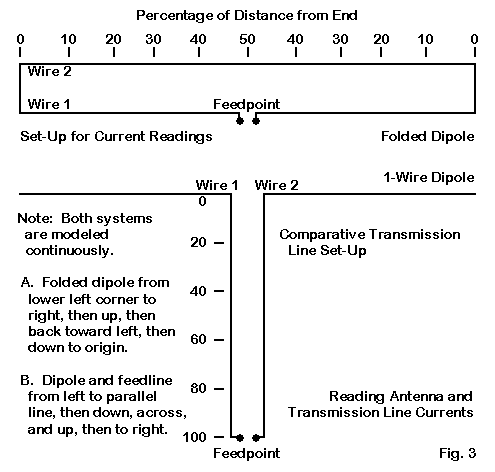To demonstrate this, we can use the model on the lower half of Fig 3 to derive the currents along the transmission line. A sample every 20% of the way of a line nearly, but not quite, 1 wavelength long is instructive. We shall present two sets of current phase figures for wire #2: onset derived from the modeling convention of continuously developing the model from left to right, the other from using the dipole junction as the starting point for both wires.

```     Currents            Wire 1                        Wire 2
Distance       Magnitude      Phase          Magnitude      Phase
0%           1.001          -  0.1         1.001          -  0.0/ 180.0
20%           0.419            18.1         0.419            18.1/-162.0
40%           0.758           173.5         0.758           173.5/-  6.5
60%           0.861          -174.6         0.861          -174.6/   5.4
80%           0.270          - 31.1         0.270          - 31.0/ 148.8
100%           0.999          -  0.2         0.999          -  0.2/ 179.8```

The modeling convention that runs the transmission line wires in opposite directions shows essentially the same values for each point on the line. The convention that starts and ends them in the same direction shows the 180-degree out-of-phase condition.

To illustrate what we actually encounter with a folded dipole, let us turn to the upper portion of Fig. 3. The markers represent percentages of distance from the outer end of each wire inward toward the center. If we plot the current magnitudes and phases for a typical folded dipole, we end up with an interesting chart. Let's use our #18 bare copper wire folded dipole with 3" spacing as a test case. Current magnitudes are relative to a maximum value of 1.0, while current phases are relative to a feedpoint value of 0.0 degrees. The first current phase figure for Wire 2 is for continuous modeling so the end 2 of one wire becomes end 1 of the next. The second value presumes a model with both parallel wires starting at the same end of the assembly.

```     Currents            Wire 1                        Wire 2
Distance       Magnitude      Phase          Magnitude      Phase
0% (end)      0.256          - 74.5         0.244          -106.4/  73.6
10%            0.463          - 33.8         0.432          -153.0/  27.0
20%            0.682          - 20.2         0.659          -166.5/  13.5
30%            0.855          - 12.4         0.842          -172.6/   7.4
40%            0.964          -  6.2         0.960          -176.1/   3.9
50%            1.000             0.0         1.000          -177.9/   2.1```

The chart ends at the antenna center point because the opposite side of the antenna shows virtually identical current values at the prescribed points. Although the current magnitudes are comparable (and would be closer had the wire been without any loss at all), the current phase values show a curious pattern. Corresponding point along the wires show similar absolute current phase values, but they are opposite in sign when both wires are modeled from the same point (e.g., left to right). The pattern is distinctly unlike a transmission line that is acting like a transmission line, even with the far end a short circuit.

A phase pattern similar to the one shown is necessary if the folded dipole is to radiate. Radiation is simply the ability of the fields that result from the current levels at each point along the antenna to expand without limit. This condition is unlike that in a transmission line, where the fields are confined such that radiation is negligible. For that condition to exist, the current magnitudes would have to be equal, and the phase values must be exactly opposite.

The upshot is that we need not treat a resonant folded dipole made of transmission line like a transmission line. We can ignore the velocity factor for the line as a line and only adjust the length according to the antenna velocity factor created by the line insulation (perhaps a percent or two shorter). The practice of shorting out a folded dipole at the point indicated by the transmission line velocity factor has never shown any evidence of doing anything but shorting out the wires at that point. Finding the resonant length of the folded dipole will be challenge enough.

### Other Impedance Values

The folded dipole is ultimately simply a dipole with an impedance transformation mechanism built into its structure. As a dipole, on its fundamental frequency, it provides all of the performance we expect from a dipole--no more and no less. It tends to have a slightly wider SWR operating bandwidth (when transformed to our feedline value) than a single wire dipole because it acts like a fat wire. But it remains in performance simply a dipole.

The impedance transformation possibilities, however, should not be overlooked. The rules of thumb for transformation more than or less than 4:1 can be useful in some contexts. Before looking at potential applications, let's first look briefly at the levels of departure from 4:1 as we systematically vary the element diameters. We shall use the 3" spacing from earlier samples, but this time, we shall run each wire through a range of 0.1 to 0.5 inches in diameter--with one wire increasing as the other decreases.

To perform the modeling for this task, we shall set aside NEC-2. NEC has a known difficulty in dealing with closely spaced wires of different diameters. Fortunately, MININEC has no such limitation and handles the calculation task with ease. WE shall list the impedance ratio calculated by the equation, the resultant feedpoint impedance, and then the modeled values. This should give us a quick view as to whether the calculations and models reliably coincide.

```--------- Calculated ---------------    --------- Modeled ----------------
Diameter  Diameter  Z Ratio   Feed Z    Length    Gain      Feed Impedance
d1         d2               R=Z Ohms  inches    dBi       R +/- jX Ohms
0.1       0.5       7.01      498       193.34    2.09      493.0 + j0.0
0.2       0.4       5.09      361       193.20    2.10      363.0 + j0.0
0.3       0.3       4.00      284       193.10    2.10      288.0 - j0.0
0.4       0.2       3.23      229       193.48    2.11      234.0 - j0.0
0.5       0.1       2.58      183       193.96    2.11      189.0 - j0.0```

Given that the calculations do not account for the end wires, the coincidence of models and calculations is excellent. Incidentally, in all models, the end wires were sized to match the smaller of the two diameters involved. Moreover, the absence of any perceptible change of gain in the series of models is notable. However we size the wires in our folded dipole, it gives us dipole performance. To at least some degree, this convention accounts for the very small differences in resonant lengths of the models.

In many beams using the dipole as a driven element, the feedpoint impedance will be far less than 70-72 Ohms. Values from 10 to 50 Ohms are common, although values above 20-25 Ohms are preferred in order to reduce power losses from the accumulation of small resistances at connections. Using a folded dipole with "designer" values for element diameters and spacing, it is possible to raise the impedance to match almost any value higher than the initial feedpoint impedance. One option is to use a low transformation ratio to arrive directly at 50 Ohms. A second option is to use a higher value to arrive at 200 Ohms and then to use a 4:1 balun at the feedpoint to return to 50 Ohms with an accompanying reduction on possible common mode currents on the coax. Although HF use of folded dipole drivers is rare, at VHF they are still very popular.

### The Folded Dipole as a Doublet

Before departing the land of folded dipoles, we should at least glance at the potential of the folded dipole as a multi-band doublet. As a sample, we can look at the performance potential of an 80-meter folded dipole. I resonated a 132.85' version at 3.5 MHz. Wire spacing is about 2' and the wire are about 0.3" in diameter. I then checked the patterns and performance on other amateur bands. Numbers are rounded, since we are looking only for suggestive results.
```Frequency      Max. Gain      Feed Impedance      Pattern
MHz            dBi          R +/- jX Ohms       No. of lobes
3.5           2.12           287 - j   1          2
7.0           2.27             5 - j 160          2
10.1           3.31           540 - j 750          6
14.0           3.34            25 - j 330          4
18.1           4.71           480 + j  15         10
21.0           4.26            85 - j 530          6
24.9           4.75           550 - j 310         14
28.0           5.03           225 - j 750          8```

The gain figures and the number of pattern lobes coincide with numbers we would obtain from a single-wire dipole pressed into multi-band doublet service. What differs is the impedance value set. The difficulty of using a folded dipole on an even harmonic of the band for which it is initially resonated lies in the very low resistive component of the feedpoint impedance. By the sixth harmonic, we have a value that, while low, is well within the capabilities of most ATUs. In contrast, the second harmonic impedance of 5 Ohms is likely beyond the reach--or at least the efficient range--of most ATUs. The 4th harmonic (20 meters in this sample) might well be matchable, depending upon ATU design.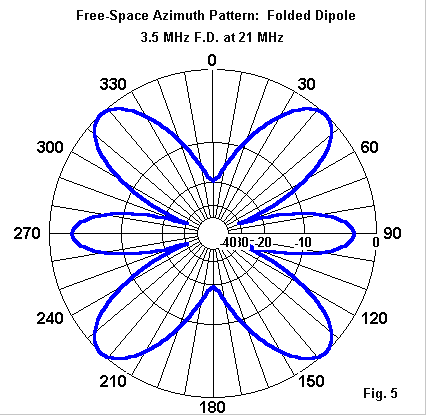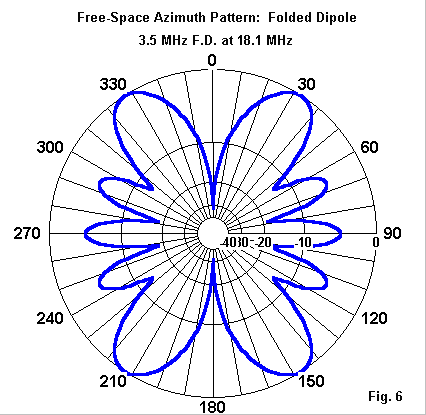Fig. 4 shows the free-space azimuth pattern for 14 MHz, with its typical 2-wavelength 4-lobe pattern. Fig. 5 presents the 6-lobe, 3 wavelength pattern for 21 MHz. The point of these figures shows up in Fig. 6, the pattern for 18.1 MHz. At about 2.5 wavelengths long, the antenna shows both the growing lobes for the 3 wavelength pattern and the diminishing lobes for the 2 wavelength pattern--for a total of 10 lobes.

Using a folded dipole as a multi-band doublet--with parallel feedline to an antenna tuner--thus becomes a matter of matching rather than of pattern development. Very low impedances may also be lossy, thus reducing performance even if a match can be obtained from a given ATU and feedline length.

For multi-band use, a folded dipole offers no advantage over a single-wire doublet of the same approximate length. Indeed, in the final analysis, perhaps the only reason for using a folded dipole is where the impedance transformation is of special interest, that is, where it may resolve an antenna design challenge. A secondary use would be to offer a path to discharge static charge build-up and thus to reduce one (of the many) noise sources. However, there are other means to this same goal.

Otherwise, the folded dipole performs just like a fat single-wire dipole.Updated 03-12-2000. © L. B. Cebik, W4RNL. Data may be used for personal purposes, but may not be reproduced for publication in print or any other medium without permission of the author.